# Basic acoustics appendices

## Basic acoustics appendices

In the following appendices you will find detailed information that supplements the material supplied in the main body. The first two appendices explain basic concepts (ie. scientific notation and logarithms) that are assumed for this course. YOU WILL NOT BE EXAMINED ON ANY MATERIAL WHICH IS ONLY FOUND IN THE APPENDICES. This material is supplied for those students who wish to have a more detailed knowledge of general acoustics over and above the requirements of this course.

### APPENDIX 1

#### Scientific Notation

Scientific notation is used to make very large or very small numbers more manageable. The principle is described below by example.

 1,000 = 103 10,000 = 104 1,000,000 = 106 2,000,000 = 2 x 106 5,430,000 = 5.43 x 106 10 = 101 1 = 100 0 = 0 0.1 = 10-1 (nb. X-Y 1/XY) 0.0001 = 10-4 0.0003 = 3 x 10-4 0.00034 = 3.4 x 10-4

### APPENDIX 2

#### Logarithms

The two most common types of logarithm are "natural" logarithms (base e) and base 10 logarithms. Only base 10 logarithms are of interest in this course as they form the basis of the deciBel.

 If 10A = B then log10B = A

In other words, determining a logarithm asks to what exponent the value 10 must be raised in order for the result to equal the value B.

 log10100 = 2 log101,000 = 3 log102 = 0.3 log102,000 = 3.3

Note that:-

• log10(A x B)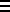log10(A) + log10(B) (eg. log10(1,000 x 2)log101,000 + log102)
• log10(A/B)log10A - log10B
• log10(AB)B x log10A

### APPENDIX 3

#### Basic Physical Units of Measurement

There are two main metric systems of measurement based on the choice of the basic units which make them up:-

MKS (Metres, Kilograms, Seconds)

CGS (Centimetre, Grams, Seconds)

There is also the British Imperial system, which has been gradually phased out over the past 50 years and which was based on the basic units:-

FPS (Feet, Pounds, Seconds)

The MKS system has now been recognised as the international system for science and has also been increasingly adopted for day-to-day use. You will, however, still come across CGS units in various books and articles on acoustics and so the various CGS measurements will also be listed below.

IN THIS COURSE, ONLY THE MKS SYSTEM IS USED

 MKS CGS Mass kilogram (kg) gram (g) Length metre (m) centimetre (cm) Time second (sec,s) second (sec,s)

Most, but not all, other physical measurements are made up of some combination of these three basic units of mass (M), length (L), and time (T).

 Area (L2) m2 cm2 Volume (L3) m3 cm3 Velocity (L/T) or LT Distance travelled per unit time. m.s-1 cm.s-1 Acceleration (L/T/T or L/T2 or LT-2) Rate at which velocity changes. m.s-2 cm.s-2 Force (MLT-2 M x acceleration) A force causes a mass to accelerate (or decelerate) kg.m.s-2 g.cm.s-2 known as Newton (N) dyne (1 dyne10-5 Newtons) Work (ML2T-2 force (F) x L [MLT-2.L]) A measure of energy transfer from one body to another. Work only occurs when there is a transfer of energy. Work can be related to the distance an object is moved (L) by a force (F). kg.m2.s-2 g.cm2.s-2 or Newton.m dyne.cm known as Joule erg (1 erg10-7 joules) Power (ML2T-3 work/T [ML2T-2 x T-1] force x velocity [MLT-2 x LT-1]) The rate at which work is done (ie. work per second). That power which gives rise to the production of energy at the rate of 1 joule per second or 107 ergs per second. kg.m2.s-3 g.cm2.s-3 or Joule.s-1 erg.s-1 known as Watt (W) ... 107 erg.s-11 Watt Intensity (MT-3 power/area [ML2T-3.L-2] work/area/sec [ML2T-2.L-2.T-1]) Rate at which work is done across a unit area per second. Power per unit area. kg.s-3 g.s-3 or Joule.m-2.s-1 erg.cm-2.s-1 or Watt.m-2 Watt.cm-2 (1 Watt.cm-2104Watt.m-2) Pressure (ML-1T-2 force/area [MLT-2.L-2]) The amount of force per unit area. kg.m-1.s-2 g.cm-1.s-2 or Newton.m-2 dyne.cm-2 known as Pascal (Pa) ...

### APPENDIX 4

#### Acoustic Units of Measurement

Acoustic units of measurement are a sub-set of the full list of units of measurement.

 Wavelength (L) m cm Frequency (T-1) Velocity (speed of sound) divided by distance (wavelength) LT-1.L-1 Hertz (Hz)cycles/secsec-1 kiloHertz (kHz)1000 Hz Acoustic Power (ML2T-3 work/T [ML2T-2 x T-1] force x velocity [MLT-2 x LT-1]) The rate at which work is done (ie. work per second) kg.m2.s-3 g.cm2.s-3 or Joule.s-1 erg.s-1 known as Watt (W) ... 107 erg.s-11 Watt Acoustic Intensity (MT-3 power/area [ML2T-3.L-2] work/area/sec [ML2T-2.L-2.T-1]) Amount of power per unit area. kg.s-3 g.s-3 or Joule.m-2.s-1 erg.cm-2.s-1 or Watt.m-2 Watt.cm-2 (1 Watt.cm-2104Watt.m-2) Acoustic or Sound Pressure (ML-1T-2 force/area [MLT-2.L-2]) The amount of force per unit area. kg.m-1.s-2 g.cm-1.s-2 or Newton.m-2 dyne.cm-2 known as Pascal (Pa) ... (1 dyne.cm-2 0.1 Pa)

Acoustic Pressure is commonly referred to as Sound Pressure Level or SPL.

### APPENDIX 5

#### Some Extra Notes on deciBels

The formula for deriving the Bel from a ratio of intensities is:-

Bel = log10(I1/I2)

Since there are 10 dB to every Bel, the formula for deriving the deciBel (dB) is:-

dB = 10 x log10(I1/I2)

The formula for determining dB from pressure ratios is derived as follows:-

Since:-

log10(X2) = 2 x log10(X)

and:-

(I1/I2) = (P1/P2)2

Then:-

log10(I/I0) = log10((P/P0)2) = 2 x log10(P/P0)

and so:-

10 x log10(I/I0) = 20 x log10(P/P0)

iff (if and only if):-

P is the pressure of a sound whose intensity is I

P0 is the pressure of a sound whose intensity is I0

The second condition is only approximately true (when using the standard reference values) as 20µPa is only approximately the pressure of a sound whose intensity is 10-12W.m-2

### APPENDIX 6

#### THE VELOCITY OF SOUND

The velocity or speed of sound (c) is the number of metres that a wavefront travels in one second.

The speed of sound in a gas is dependent on a number of factors:-

1. the composition of the gas (eg. air80% oxygen + 20% nitrogen)
2. pressure
3. temperature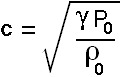cvelocity of sound P0static pressure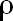0static density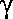ratio of specific heats

The ratio of specific heats () varies with gas and is proportional to temperature, so:-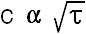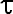absolute temperature (K: degrees Kelvin) nb. 0°C = 273.15°K (273°K), and so °K = °C + 273.

These relationships are quite complex as an increase in temperature affects both0 and P0, but under conditions where pressure and density remain constant (eg. same gas under same pressure conditions, such as air at sea level) the following relation holds:-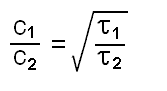To use this equation all temperature values in °C must be converted to °K (see above)

The exact velocity of sound in air at 1 atmosphere and 0C is 331 ms-1 (although this is often rounded off to 330 ms-1 to simplify calculations). This is the same as saying that the velocity of sound at 273°K is 331 ms-1, and for air at the same pressure but different temperatures, the velocity of sound can be calculated by the above formula (nb. if the gas changes, or if the pressure changes, then the calculations will be inaccurate). Using this formula it can be shown that for air at 1 atmosphere pressure:-

if= 293°K (20°C) then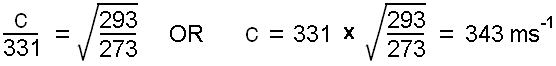Similarly:-

if = 298°K (25°C) then c = 346 ms-1

if = 303°K (30°C) then c = 349 ms-1

The following table compares the velocity of sound and the density of three gasses at 0°C and 1 atmosphere:-

 Gas0 c Hydrogen 0.09 1286 Oxygen 1.4 317 Air 1.3 331

It can be clearly seen from this table that the velocity of sound in very low density gasses (such as hydrogen) tends to be higher than it is in higher density gasses (such as oxygen and air). The main cause of the difference in the velocity of sound in oxygen and air would be the effect that the density of nitrogen has on the density of air (a mix of 20% oxygen and 80% nitrogen).

The velocity of sound in liquids and solids depends upon the elasticity and density.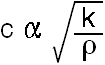density kelastic or bulk modulus

It must be noted that the elastic or bulk modulus increases as the compressibility or deformability of the material decreases, so the elastic or bulk modulus is a measure of the resistance of a material to deformation. That is, the elastic or bulk modulus is the inverse of the common notion of elasticity and so the lower k the higher the elasticity. In other words, increases in either elasticity (decrease in k) or in density lead to a decrease in the speed of sound.

The following table indicates some relationships between density and velocity of sound for various solids and liquids:-

 Material Temp (°C) Density (x 103 kgm-3) c (ms-1) Water 15 1.0 1450 vulcanised rubber 0 1.1 54 granite 0 2.7 6000 aluminium 20 2.7 5100 iron 20 7.9 5130 copper 20 8.9 3560 lead 20 11.3 1230

The table can be interpreted if it is realised that the compressibility of the metals copper, iron, and lead is fairly similar so that the main trend in these metals is a decrease in the velocity of sound as the density increases. Aluminium and iron on the other hand have very different densities but sound travels through them at about the same velocity. If they were equally compressible then sound would travel at a much higher velocity through lower density aluminium. The fact that it does not indicates that the velocity of sound in aluminium is reduced because it is more compressible (lower bulk modulus) than iron. Granite, on the other hand has the same density as aluminium but sound travels at a greater velocity because its compressibility is less (higher bulk modulus). Water has a very low density, but sound has a similar velocity to what it has in lead. This suggests that water is much more compressible than lead (has a lower bulk modulus). Vulcanised rubber is extremely compressible (very low bulk modulus) and so, regardless of its low average density, sound travels very slowly.

### APPENDIX 7

#### ACOUSTIC INTENSITY AND THE INVERSE SQUARE LAW

The acoustic intensity, or average rate at which work is being transferred through a unit area (on the surface of the spherical wave front radiating out from the source in all directions) diminishes with distance in accordance with the inverse square law.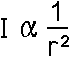That is, if you multiply the distance from the source by two, you divide the intensity by four. This law is derived from:-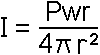where:- Iintensity of the sound per unit area at distance r from the source Pwrtotal power of the sound rradius of sphere (distance from sound source) 4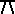r2surface area of a sphere of radius r

For the purposes of this course, we are more interested in comparing intensities (and pressures) at varying distances from the sound source. From the first equation above we can derive:-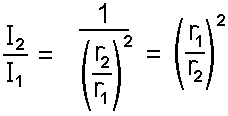or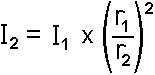where:- I1 the intensity of a sound at distance r1 from the source I2 the intensity of the same sound at distance r2 from the source.

If we remember that:-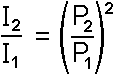then we can also say:-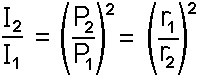or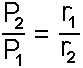In other words:-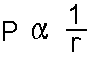That is sound pressure is inversely proportional to the distance of the point of measurement from the source, so that if you double the distance you halve the sound pressure.

Content owner: Department of Linguistics Last updated: 12 Mar 2020 12:26pm